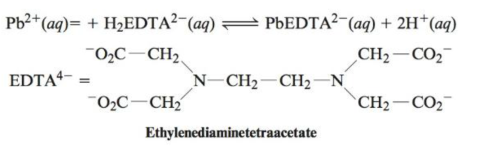# EDTA is used as a complexing agent in chemical analysis. Solutions of EDTA, usually containing the disodium salt Na 2 H 2 EDTA, are also used to treat heavy metal poisoning. The equilibrium constant for the following reaction is 6.7 × 10 21 : Calculate [Pb 2+ ] at equilibrium in a solution originally 0.0050 M in Pb 2+ , 0.075 M in H 2 EDTA 2− , and buffered at pH = 7.00.### Chemistry: An Atoms First Approach

2nd Edition
Steven S. Zumdahl + 1 other
Publisher: Cengage Learning
ISBN: 9781305079243

#### Solutions

Chapter
Section### Chemistry: An Atoms First Approach

2nd Edition
Steven S. Zumdahl + 1 other
Publisher: Cengage Learning
ISBN: 9781305079243
Chapter 19, Problem 78AE
Textbook Problem
15 views

## EDTA is used as a complexing agent in chemical analysis. Solutions of EDTA, usually containing the disodium salt Na2H2EDTA, are also used to treat heavy metal poisoning. The equilibrium constant for the following reaction is 6.7 × 1021:Calculate [Pb2+] at equilibrium in a solution originally 0.0050 M in Pb2+, 0.075 M in H2EDTA2−, and buffered at pH = 7.00.

Interpretation Introduction

Interpretation: The equilibrium constant of a reaction, pH value of buffer solution, concentration of solution in Pb2+ and concentration of solution in H2EDTA2 is given. The concentration of Pb2+ at equilibrium is to be calculated.

Concept introduction: At equilibrium, the equilibrium constant expression is expressed by the formula,

K=ConcentrationofproductsConcentrationofreactants

To determine: The concentration of Pb2+ at equilibrium for the given reaction.

### Explanation of Solution

Explanation

The [H+] is 1×107M_ .

Given

The pH value is 6.00 .

Formula

The concentration of H+ is calculated using the formula,

pH=log10[H+]

Substitute the value of pH in the above equation.

pH=log10[H+]7.00=log10[H+][H+]=1×107M_

The concentration of Pb2+ at equilibrium for the given reaction is 8.6×105M_ .

Given

The value of K is 6.7×1021 .

Concentration of solution in Pb2+ is 0.0050M .

Concentration of solution in H2EDTA2 is 0.075M .

The given reaction is,

Pb2+(s)+H2EDTA2(aq)PbEDTA2(aq)+2H+(aq)

The ICE table for the stated reaction is formed.

Pb2+(aq)+H2EDTA2(aq)PbEDTA(aq)+2H+(aq)Initial(M):         0.00500.07000Change(M)      xx+x+2xEquilibrium(M):0

### Still sussing out bartleby?

Check out a sample textbook solution.

See a sample solution

#### The Solution to Your Study Problems

Bartleby provides explanations to thousands of textbook problems written by our experts, many with advanced degrees!

Get Started